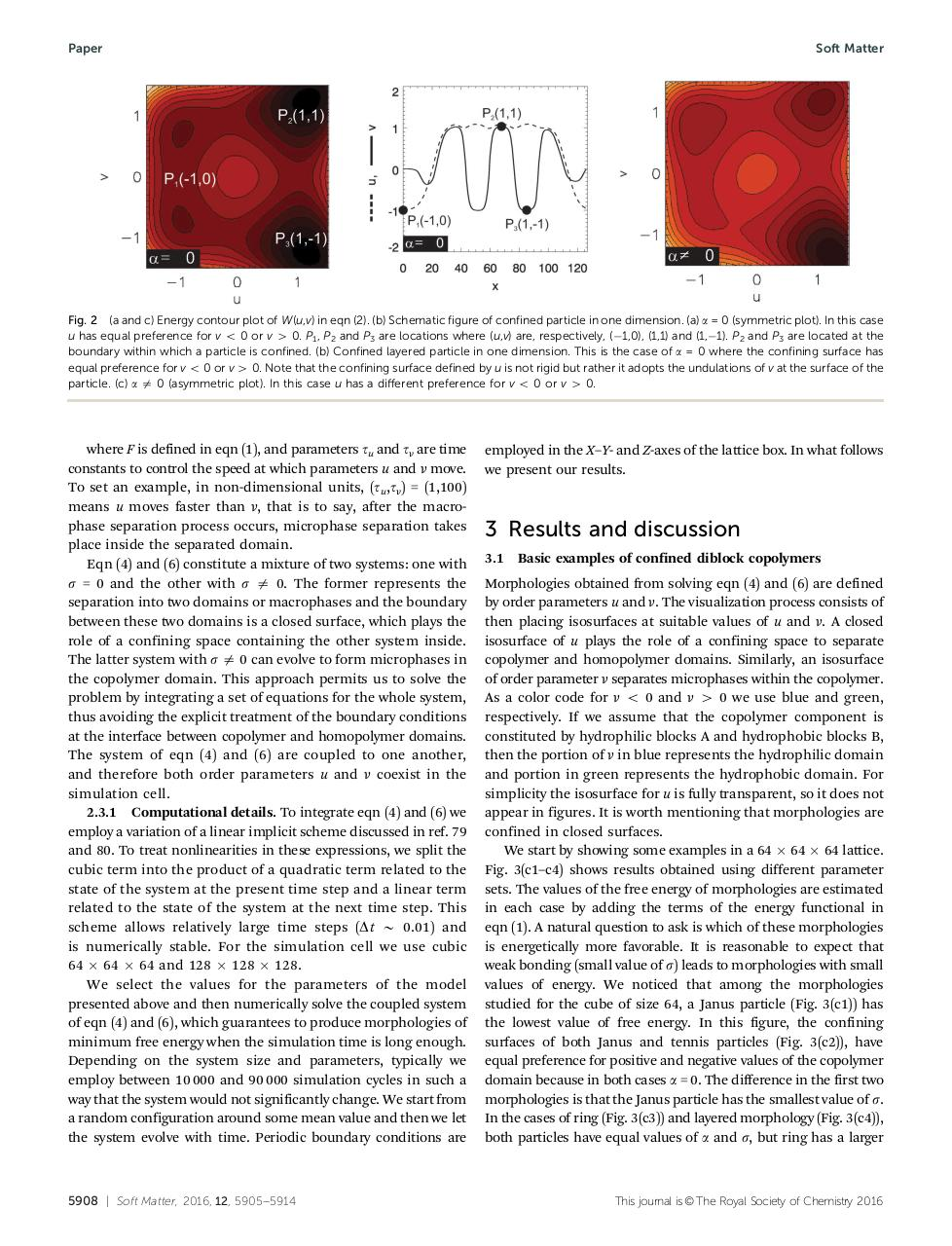# DBCPsoftmatter2016.pdfPage 1 2 3 4 5 6 7 8 9 10

#### Text preview

Paper

Soft Matter

Fig. 2 (a and c) Energy contour plot of W(u,v) in eqn (2). (b) Schematic figure of confined particle in one dimension. (a) a = 0 (symmetric plot). In this case
u has equal preference for v o 0 or v 4 0. P1, P2 and P3 are locations where (u,v) are, respectively, (1,0), (1,1) and (1,1). P2 and P3 are located at the
boundary within which a particle is confined. (b) Confined layered particle in one dimension. This is the case of a = 0 where the confining surface has
equal preference for v o 0 or v 4 0. Note that the confining surface defined by u is not rigid but rather it adopts the undulations of v at the surface of the
particle. (c) a a 0 (asymmetric plot). In this case u has a diﬀerent preference for v o 0 or v 4 0.

where F is defined in eqn (1), and parameters tu and tv are time
constants to control the speed at which parameters u and v move.
To set an example, in non-dimensional units, (tu,tv) = (1,100)
means u moves faster than v, that is to say, after the macrophase separation process occurs, microphase separation takes
place inside the separated domain.
Eqn (4) and (6) constitute a mixture of two systems: one with
s = 0 and the other with s a 0. The former represents the
separation into two domains or macrophases and the boundary
between these two domains is a closed surface, which plays the
role of a confining space containing the other system inside.
The latter system with s a 0 can evolve to form microphases in
the copolymer domain. This approach permits us to solve the
problem by integrating a set of equations for the whole system,
thus avoiding the explicit treatment of the boundary conditions
at the interface between copolymer and homopolymer domains.
The system of eqn (4) and (6) are coupled to one another,
and therefore both order parameters u and v coexist in the
simulation cell.
2.3.1 Computational details. To integrate eqn (4) and (6) we
employ a variation of a linear implicit scheme discussed in ref. 79
and 80. To treat nonlinearities in these expressions, we split the
cubic term into the product of a quadratic term related to the
state of the system at the present time step and a linear term
related to the state of the system at the next time step. This
scheme allows relatively large time steps (Dt B 0.01) and
is numerically stable. For the simulation cell we use cubic
64  64  64 and 128  128  128.
We select the values for the parameters of the model
presented above and then numerically solve the coupled system
of eqn (4) and (6), which guarantees to produce morphologies of
minimum free energy when the simulation time is long enough.
Depending on the system size and parameters, typically we
employ between 10 000 and 90 000 simulation cycles in such a
way that the system would not significantly change. We start from
a random configuration around some mean value and then we let
the system evolve with time. Periodic boundary conditions are

5908 | Soft Matter, 2016, 12, 5905--5914

employed in the X–Y- and Z-axes of the lattice box. In what follows
we present our results.

3 Results and discussion
3.1

Basic examples of confined diblock copolymers

Morphologies obtained from solving eqn (4) and (6) are defined
by order parameters u and v. The visualization process consists of
then placing isosurfaces at suitable values of u and v. A closed
isosurface of u plays the role of a confining space to separate
copolymer and homopolymer domains. Similarly, an isosurface
of order parameter v separates microphases within the copolymer.
As a color code for v o 0 and v 4 0 we use blue and green,
respectively. If we assume that the copolymer component is
constituted by hydrophilic blocks A and hydrophobic blocks B,
then the portion of v in blue represents the hydrophilic domain
and portion in green represents the hydrophobic domain. For
simplicity the isosurface for u is fully transparent, so it does not
appear in figures. It is worth mentioning that morphologies are
confined in closed surfaces.
We start by showing some examples in a 64  64  64 lattice.
Fig. 3(c1–c4) shows results obtained using different parameter
sets. The values of the free energy of morphologies are estimated
in each case by adding the terms of the energy functional in
eqn (1). A natural question to ask is which of these morphologies
is energetically more favorable. It is reasonable to expect that
weak bonding (small value of s) leads to morphologies with small
values of energy. We noticed that among the morphologies
studied for the cube of size 64, a Janus particle (Fig. 3(c1)) has
the lowest value of free energy. In this figure, the confining
surfaces of both Janus and tennis particles (Fig. 3(c2)), have
equal preference for positive and negative values of the copolymer
domain because in both cases a = 0. The difference in the first two
morphologies is that the Janus particle has the smallest value of s.
In the cases of ring (Fig. 3(c3)) and layered morphology (Fig. 3(c4)),
both particles have equal values of a and s, but ring has a larger

This journal is © The Royal Society of Chemistry 2016